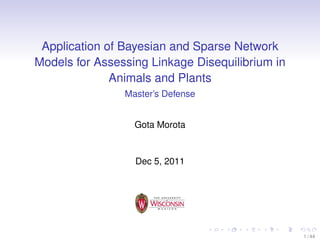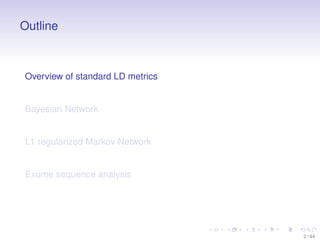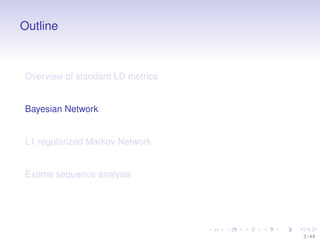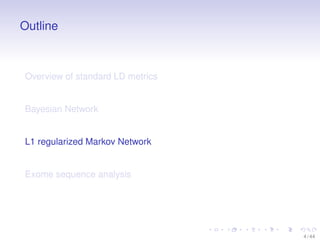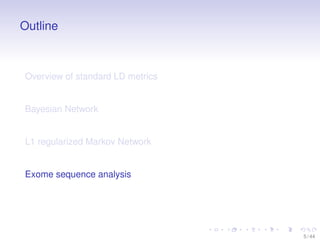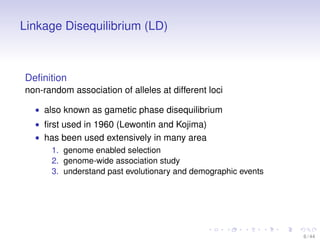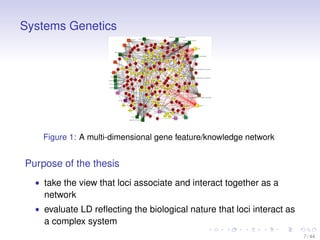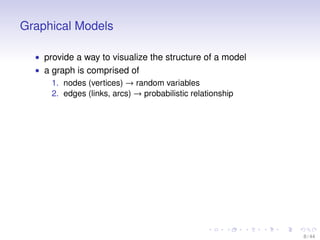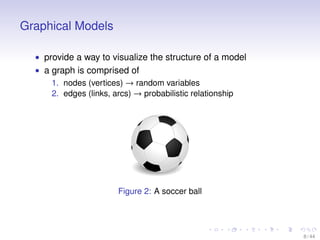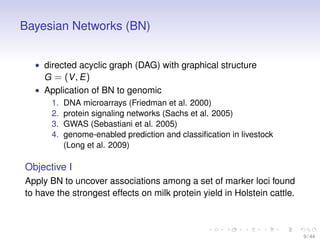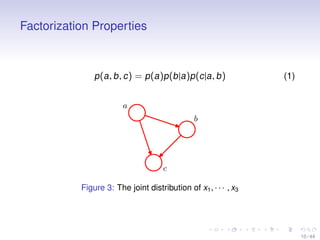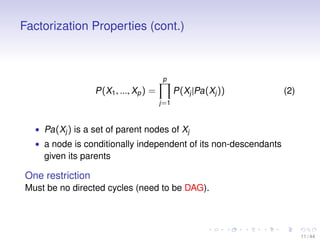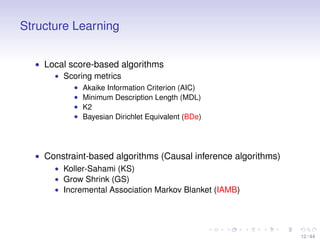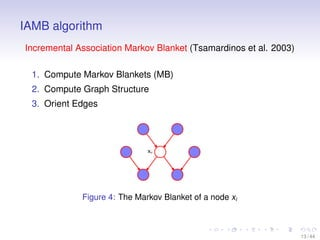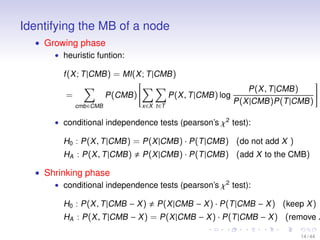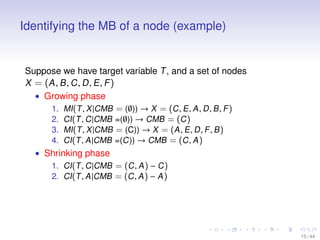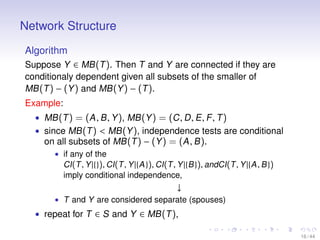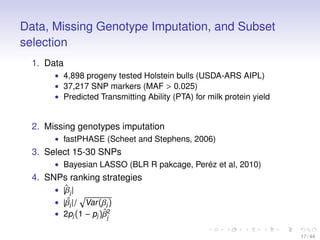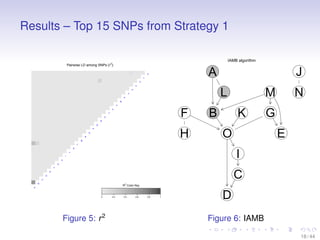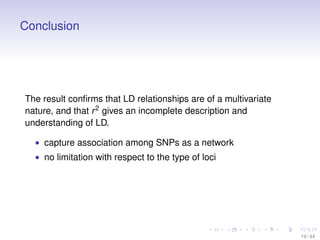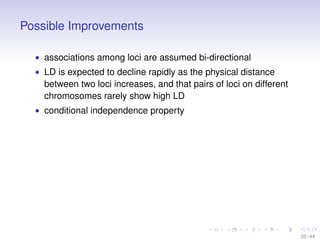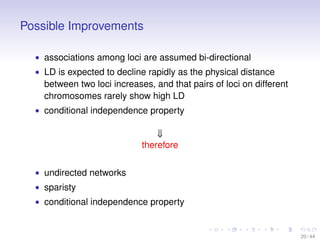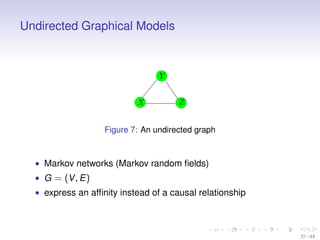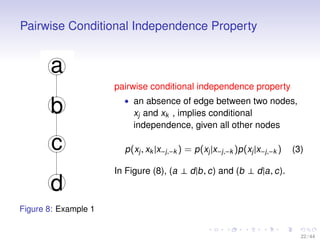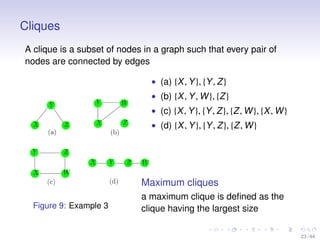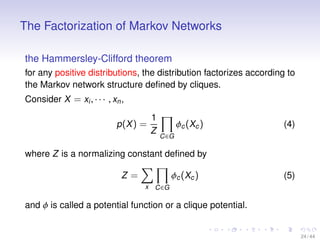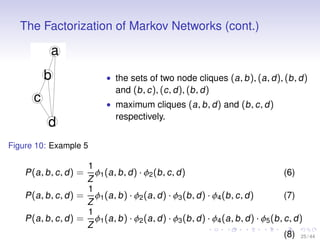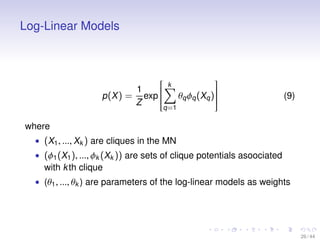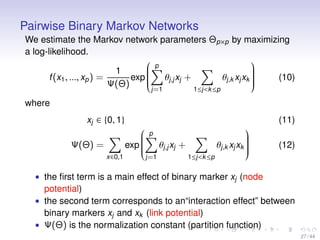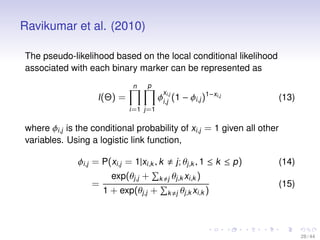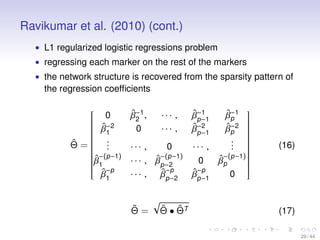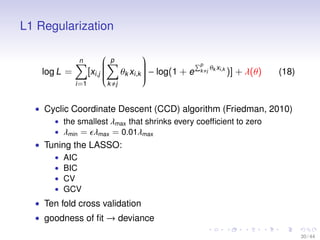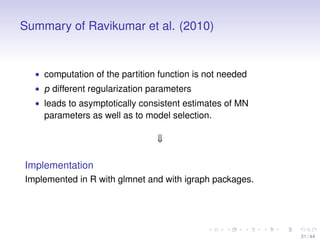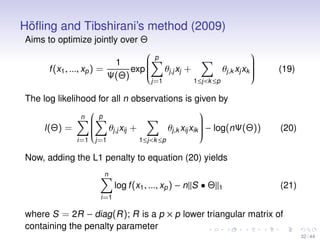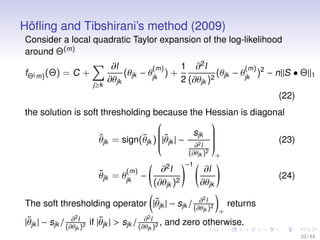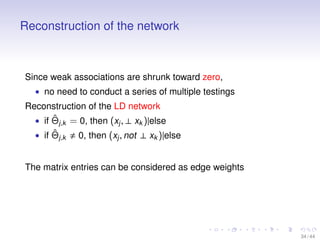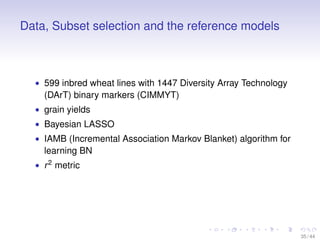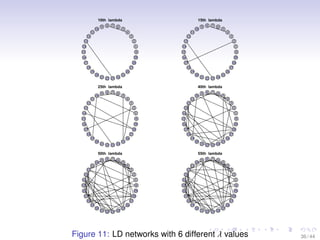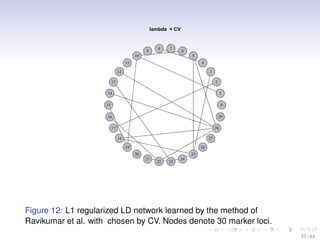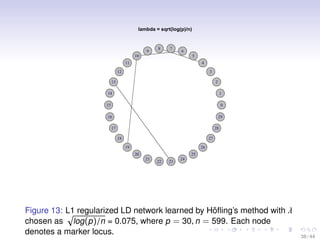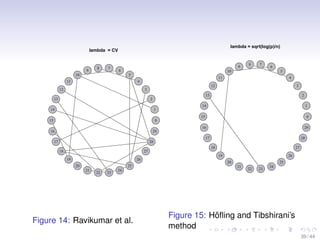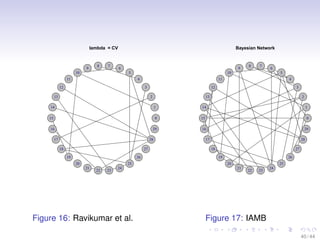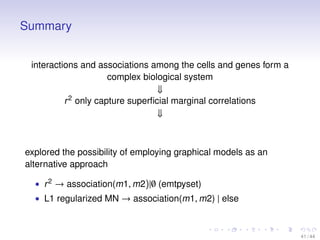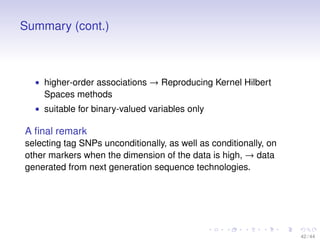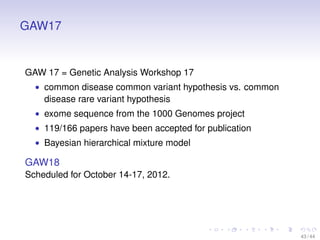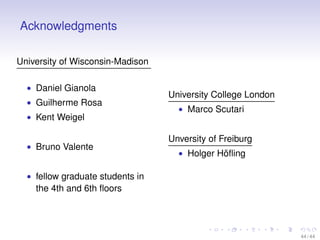1 of 46

### Application of Bayesian and Sparse Network Models for Assessing Linkage Disequilibrium in Animals and Plants

1. Application of Bayesian and Sparse Network Models for Assessing Linkage Disequilibrium in Animals and Plants Master’s Defense Gota Morota Dec 5, 2011 1 / 44
2. Outline Overview of standard LD metrics Bayesian Network L1 regularized Markov Network Exome sequence analysis 2 / 44
3. Outline Overview of standard LD metrics Bayesian Network L1 regularized Markov Network Exome sequence analysis 3 / 44
4. Outline Overview of standard LD metrics Bayesian Network L1 regularized Markov Network Exome sequence analysis 4 / 44
5. Outline Overview of standard LD metrics Bayesian Network L1 regularized Markov Network Exome sequence analysis 5 / 44
6. Linkage Disequilibrium (LD) Deﬁnition non-random association of alleles at different loci • also known as gametic phase disequilibrium • ﬁrst used in 1960 (Lewontin and Kojima) • has been used extensively in many area 1. genome enabled selection 2. genome-wide association study 3. understand past evolutionary and demographic events 6 / 44
7. Systems Genetics Figure 1: A multi-dimensional gene feature/knowledge network Purpose of the thesis • take the view that loci associate and interact together as a network • evaluate LD reﬂecting the biological nature that loci interact as a complex system 7 / 44
8. Graphical Models • provide a way to visualize the structure of a model • a graph is comprised of 1. nodes (vertices) → random variables 2. edges (links, arcs) → probabilistic relationship 8 / 44
9. Graphical Models • provide a way to visualize the structure of a model • a graph is comprised of 1. nodes (vertices) → random variables 2. edges (links, arcs) → probabilistic relationship Figure 2: A soccer ball 8 / 44
10. Bayesian Networks (BN) • directed acyclic graph (DAG) with graphical structure G = (V , E ) • Application of BN to genomic 1. DNA microarrays (Friedman et al. 2000) 2. protein signaling networks (Sachs et al. 2005) 3. GWAS (Sebastiani et al. 2005) 4. genome-enabled prediction and classiﬁcation in livestock (Long et al. 2009) Objective I Apply BN to uncover associations among a set of marker loci found to have the strongest effects on milk protein yield in Holstein cattle. 9 / 44
11. Factorization Properties p (a , b , c ) = p (a )p (b |a )p (c |a , b ) (1) Figure 3: The joint distribution of x1 , · · · , x3 10 / 44
12. Factorization Properties (cont.) p P (X1 , ..., Xp ) = P (Xj |Pa (Xj )) (2) j =1 • Pa (Xj ) is a set of parent nodes of Xj • a node is conditionally independent of its non-descendants given its parents One restriction Must be no directed cycles (need to be DAG). 11 / 44
13. Structure Learning • Local score-based algorithms • Scoring metrics • • • • Akaike Information Criterion (AIC) Minimum Description Length (MDL) K2 Bayesian Dirichlet Equivalent (BDe) • Constraint-based algorithms (Causal inference algorithms) • Koller-Sahami (KS) • Grow Shrink (GS) • Incremental Association Markov Blanket (IAMB) 12 / 44
14. IAMB algorithm Incremental Association Markov Blanket (Tsamardinos et al. 2003) 1. Compute Markov Blankets (MB) 2. Compute Graph Structure 3. Orient Edges Figure 4: The Markov Blanket of a node xi 13 / 44
15. Identifying the MB of a node • Growing phase • heuristic funtion: f (X ; T |CMB ) = MI(X ; T |CMB ) = cmb ∈CMB     P (CMB )     P (X , T |CMB )    P (X , T |CMB ) log  P (X |CMB )P (T |CMB )   x ∈X t ∈T • conditional independence tests (pearson’s χ2 test): H0 : P (X , T |CMB ) = P (X |CMB ) · P (T |CMB ) (do not add X ) HA : P (X , T |CMB ) P (X |CMB ) · P (T |CMB ) (add X to the CMB) • Shrinking phase • conditional independence tests (pearson’s χ2 test): H0 : P (X , T |CMB − X ) P (X |CMB − X ) · P (T |CMB − X ) (keep X ) HA : P (X , T |CMB − X ) = P (X |CMB − X ) · P (T |CMB − X ) (remove X 14 / 44
16. Identifying the MB of a node (example) Suppose we have target variable T , and a set of nodes X = (A , B , C , D , E , F ) • Growing phase 1. MI(T , X |CMB = (∅)) → X = (C , E , A , D , B , F ) 2. CI(T , C |CMB =(∅)) → CMB = (C ) 3. MI(T , X |CMB = (C)) → X = (A , E , D , F , B ) 4. CI(T , A |CMB =(C)) → CMB = (C , A ) • Shrinking phase 1. CI(T , C |CMB = (C , A ) − C ) 2. CI(T , A |CMB = (C , A ) − A ) 15 / 44
17. Network Structure Algorithm Suppose Y ∈ MB (T ). Then T and Y are connected if they are conditionaly dependent given all subsets of the smaller of MB (T ) − (Y ) and MB (Y ) − (T ). Example: • MB (T ) = (A , B , Y ), MB (Y ) = (C , D , E , F , T ) • since MB (T ) < MB (Y ), independence tests are conditional on all subsets of MB (T ) − (Y ) = (A , B ). • if any of the CI(T , Y |{}), CI(T , Y |{A }), CI(T , Y |{B }), andCI(T , Y |{A , B }) imply conditional independence, ↓ • T and Y are considered separate (spouses) • repeat for T ∈ S and Y ∈ MB (T ), 16 / 44
18. Data, Missing Genotype Imputation, and Subset selection 1. Data • 4,898 progeny tested Holstein bulls (USDA-ARS AIPL) • 37,217 SNP markers (MAF > 0.025) • Predicted Transmitting Ability (PTA) for milk protein yield 2. Missing genotypes imputation • fastPHASE (Scheet and Stephens, 2006) 3. Select 15-30 SNPs • Bayesian LASSO (BLR R pakcage, Perez et al, 2010) ´ 4. SNPs ranking strategies ˆ • |βj | ˆ • |βj |/ Var (βj ) ˆ • 2pj (1 − pj )β2 j 17 / 44
19. Results – Top 15 SNPs from Strategy 1 IAMB algorithm Pairwise LD among SNPs (r2) J d A c b a Z L Y X M N W V U F T S B K G R Q P H O N O E M L K J I I H G F E C D C B R2 Color Key A 0 Figure 5: r 2 0.2 0.4 0.6 0.8 1 D Figure 6: IAMB 18 / 44
20. Conclusion The result conﬁrms that LD relationships are of a multivariate nature, and that r 2 gives an incomplete description and understanding of LD. • capture association among SNPs as a network • no limitation with respect to the type of loci 19 / 44
21. Possible Improvements • associations among loci are assumed bi-directional • LD is expected to decline rapidly as the physical distance between two loci increases, and that pairs of loci on different chromosomes rarely show high LD • conditional independence property 20 / 44
22. Possible Improvements • associations among loci are assumed bi-directional • LD is expected to decline rapidly as the physical distance between two loci increases, and that pairs of loci on different chromosomes rarely show high LD • conditional independence property ⇓ therefore • undirected networks • sparisty • conditional independence property 20 / 44
23. Undirected Graphical Models Figure 7: An undirected graph • Markov networks (Markov random ﬁelds) • G = (V , E ) • express an afﬁnity instead of a causal relationship 21 / 44
24. Pairwise Conditional Independence Property pairwise conditional independence property • an absence of edge between two nodes, xj and xk , implies conditional independence, given all other nodes p (xj , xk |x−j ,−k ) = p (xj |x−j ,−k )p (xj |x−j ,−k ) (3) In Figure (8), (a ⊥ d |b , c) and (b ⊥ d |a , c). Figure 8: Example 1 22 / 44
25. Cliques A clique is a subset of nodes in a graph such that every pair of nodes are connected by edges • (a) {X , Y }, {Y , Z } • (b) {X , Y , W }, {Z } • (c) {X , Y }, {Y , Z }, {Z , W }, {X , W } • (d) {X , Y }, {Y , Z }, {Z , W } Maximum cliques Figure 9: Example 3 a maximum clique is deﬁned as the clique having the largest size 23 / 44
26. The Factorization of Markov Networks the Hammersley-Clifford theorem for any positive distributions, the distribution factorizes according to the Markov network structure deﬁned by cliques. Consider X = xi , · · · , xn , p (X ) = 1 Z φc (Xc ) (4) C ∈G where Z is a normalizing constant deﬁned by φc (Xc ) Z= (5) x C ∈G and φ is called a potential function or a clique potential. 24 / 44
27. The Factorization of Markov Networks (cont.) • the sets of two node cliques (a , b ), (a , d ), (b , d ) and (b , c ), (c , d ), (b , d ) • maximum cliques (a , b , d ) and (b , c , d ) respectively. Figure 10: Example 5 1 φ1 (a , b , d ) · φ2 (b , c , d ) (6) Z 1 (7) P (a , b , c , d ) = φ1 (a , b ) · φ2 (a , d ) · φ3 (b , d ) · φ4 (b , c , d ) Z 1 P (a , b , c , d ) = φ1 (a , b ) · φ2 (a , d ) · φ3 (b , d ) · φ4 (a , b , d ) · φ5 (b , c , d ) Z (8) 25 / 44 P (a , b , c , d ) =
28. Log-Linear Models  k      1     θq φq (Xq ) p (X ) = exp        Z q =1 (9) where • (X1 , ..., Xk ) are cliques in the MN • (φ1 (X1 ), ..., φk (Xk )) are sets of clique potentials asoociated with k th clique • (θ1 , ..., θk ) are parameters of the log-linear models as weights 26 / 44
29. Pairwise Binary Markov Networks We estimate the Markov network parameters Θp ×p by maximizing a log-likelihood.      f (x1 , ..., xp ) = exp     Ψ(Θ) p 1 θj ,j xj + j =1 1 ≤j <k ≤p      θj ,k xj xk     (10) where xj ∈ {0, 1} Ψ(Θ) = x ∈0 , 1 (11)      exp     p θj ,j xj + j =1 1 ≤j <k ≤p      θj ,k xj xk     (12) • the ﬁrst term is a main effect of binary marker xj (node potential) • the second term corresponds to an“interaction effect” between binary markers xj and xk (link potential) • Ψ(Θ) is the normalization constant (partition function) 27 / 44
30. Ravikumar et al. (2010) The pseudo-likelihood based on the local conditional likelihood associated with each binary marker can be represented as n p x φi ,ij,j (1 − φi ,j )1−xi,j l (Θ) = (13) i =1 j =1 where φi ,j is the conditional probability of xi ,j = 1 given all other variables. Using a logistic link function, φi ,j = P(xi ,j = 1|xi ,k , k j ; θj ,k , 1 ≤ k ≤ p ) exp(θj ,j + k j θj ,k xi ,k ) = 1 + exp(θj ,j + k j θj ,k xi ,k ) (14) (15) 28 / 44
31. Ravikumar et al. (2010) (cont.) • L1 regularized logistic regressions problem • regressing each marker on the rest of the markers • the network structure is recovered from the sparsity pattern of the regression coefﬁcients   0    ˆ−2  β   1     ˆ  .  . Θ= .    −(p −1)  ˆ β   1   −p  ˆ β1 ˆ β −1 , 2 0 ··· , ··· , ··· , 0 ˆ−(p −1) · · · , β p −2 ˆ p · · · , β−−2 p ˜ Θ= ˆ ˆ Θ • ΘT ˆ 1 β−−1 p ˆ 2 β−−1 p ˆp β −1 ˆp β −2 . . .                ··· ,     −(p −1)   ˆp  0 β     −p ˆ β p −1 0 (16) (17) 29 / 44
32. L1 Regularization n log L = i =1      [xi ,j     p k j      θk xi ,k  − log(1 + e    p θ x k j k i ,k )] + λ(θ) (18) • Cyclic Coordinate Descent (CCD) algorithm (Friedman, 2010) • the smallest λmax that shrinks every coefﬁcient to zero • λmin = λmax = 0.01λmax • Tuning the LASSO: • AIC • BIC • CV • GCV • Ten fold cross validation • goodness of ﬁt → deviance 30 / 44
33. Summary of Ravikumar et al. (2010) • computation of the partition function is not needed • p different regularization parameters • leads to asymptotically consistent estimates of MN parameters as well as to model selection. ⇓ Implementation Implemented in R with glmnet and with igraph packages. 31 / 44
34. ¨ Hoﬂing and Tibshirani’s method (2009) Aims to optimize jointly over Θ      f (x1 , ..., xp ) = exp     Ψ(Θ) 1 p θj ,j xj + j =1 1 ≤j <k ≤p      θj ,k xj xk     (19) The log likelihood for all n observations is given by n l (Θ) = i =1          p θj ,j xij + j =1 1 ≤j <k ≤p      θj ,k xij xik  − log(nΨ(Θ))    (20) Now, adding the L1 penalty to equation (20) yields n log f (x1 , ..., xp ) − n||S • Θ||1 (21) i =1 where S = 2R − diag (R ); R is a p × p lower triangular matrix of containing the penalty parameter 32 / 44
35. ¨ Hoﬂing and Tibshirani’s method (2009) Consider a local quadratic Taylor expansion of the log-likelihood around Θ(m) fΘ( m) (Θ) = C + j ≥k ∂l 1 ∂2 l (m ) (m) (θjk − θjk ) + (θ − θjk )2 − n||S • Θ||1 2 jk ∂θjk 2 (∂θjk ) (22) the solution is soft thresholding because the Hessian is diagonal     sjk ˆ ˜ ˜  θjk = sign(θjk ) |θjk | − 2   ∂ l  (∂θjk ) ∂ l (∂θjk )2 2 (m ) ˜ θjk = θjk − −1           2 jk ∂ ˜ |θjk | − sjk / (∂θ l 2 jk ) ∂ ˜ if |θjk | > sjk / (∂θ 2 l 2 jk ) + ∂l ∂θjk ∂2 ˜ The soft thresholding operator |θjk | − sjk / (∂θ l)2 2 (23) (24) returns + , and zero otherwise. 33 / 44
36. Reconstruction of the network Since weak associations are shrunk toward zero, • no need to conduct a series of multiple testings Reconstruction of the LD network ˆ • if Θj ,k = 0, then (xj , ⊥ xk )|else ˆ • if Θj ,k 0, then (xj , not ⊥ xk )|else The matrix entries can be considered as edge weights 34 / 44
37. Data, Subset selection and the reference models • 599 inbred wheat lines with 1447 Diversity Array Technology (DArT) binary markers (CIMMYT) • grain yields • Bayesian LASSO • IAMB (Incremental Association Markov Blanket) algorithm for learning BN • r 2 metric 35 / 44
38. 10th lambda 15th lambda 8 7 6 9 qqq 10 5 qq q4 11 q q 3 12 q q 13 2 q q 14 1 q q 0 15 q q 29 16 q q 28 17 q q 18 27 q q 19 26 q 20 q 25 q q 22 23 q q 21 q q 24 8 7 6 9 qqq 10 5 qq q4 11 q q 3 12 q q 13 2 q q 14 1 q q 0 15 q q 29 16 q q 28 17 q q 18 27 q q 19 26 q 20 q 25 q q 22 23 q q 21 q q 24 25th lambda 40th lambda 8 7 6 9 qqq 10 5 qq q4 11 q q 3 12 q q 13 2 q q 14 1 q q 0 15 q q 29 16 q q 28 17 q q 18 27 q q 19 26 q 20 q 25 q q 22 23 q q 21 q q 24 8 7 6 9 qqq 10 5 qq q4 11 q q 3 12 q q 13 2 q q 14 1 q q 0 15 q q 29 16 q q 28 17 q q 18 27 q q 19 26 q 20 q 25 q q 22 23 q q 21 q q 24 50th lambda 55th lambda 8 7 6 9 qqq 10 5 qq q4 11 q q 3 12 q q 13 2 q q 14 1 q q 0 15 q q 29 16 q q 28 17 q q 18 27 q q 19 26 q 20 q 25 q q 22 23 q q 21 24 qq 8 7 6 9 qqq 10 5 qq q4 11 q q 3 12 q q 13 2 q q 14 1 q q 0 15 q q 29 16 q q 28 17 q q 18 27 q q 19 26 q 20 q 25 q q 22 23 q q 21 24 qq Figure 11: LD networks with 6 different λ values 36 / 44
39. lambda = CV 9 8 7 6 10 5 11 4 3 12 13 2 14 1 15 0 16 29 28 17 18 27 19 26 20 25 21 22 23 24 Figure 12: L1 regularized LD network learned by the method of Ravikumar et al. with chosen by CV. Nodes denote 30 marker loci. 37 / 44
40. lambda = sqrt(log(p)/n) 9 8 7 6 10 5 11 4 3 12 13 2 14 1 15 0 16 29 28 17 18 27 19 26 20 25 21 22 23 24 ¨ Figure 13: L1 regularized LD network learned by Hoﬂing’s method with λ chosen as log (p )/n = 0.075, where p = 30, n = 599. Each node denotes a marker locus. 38 / 44
41. lambda = sqrt(log(p)/n) lambda = CV 9 8 7 9 6 10 8 7 6 10 5 11 5 11 4 4 3 12 3 12 13 13 2 2 14 14 1 1 15 0 16 29 0 15 29 16 28 17 28 17 18 18 27 27 19 19 26 20 25 21 22 23 24 Figure 14: Ravikumar et al. 26 20 25 21 22 23 24 ¨ Figure 15: Hoﬂing and Tibshirani’s method 39 / 44
42. lambda = CV 9 8 7 Bayesian Network 9 6 10 8 7 6 10 5 11 4 3 12 5 11 13 4 3 12 13 2 2 14 1 14 15 0 15 0 16 29 16 29 28 17 18 27 19 26 20 25 21 22 23 24 Figure 16: Ravikumar et al. 1 28 17 18 27 19 26 20 25 21 22 23 24 Figure 17: IAMB 40 / 44
43. Summary interactions and associations among the cells and genes form a complex biological system ⇓ r 2 only capture superﬁcial marginal correlations ⇓ explored the possibility of employing graphical models as an alternative approach • r 2 → association(m1, m2)|∅ (emtpyset) • L1 regularized MN → association(m1, m2) | else 41 / 44
44. Summary (cont.) • higher-order associations → Reproducing Kernel Hilbert Spaces methods • suitable for binary-valued variables only A ﬁnal remark selecting tag SNPs unconditionally, as well as conditionally, on other markers when the dimension of the data is high, → data generated from next generation sequence technologies. 42 / 44
45. GAW17 GAW 17 = Genetic Analysis Workshop 17 • common disease common variant hypothesis vs. common disease rare variant hypothesis • exome sequence from the 1000 Genomes project • 119/166 papers have been accepted for publication • Bayesian hierarchical mixture model GAW18 Scheduled for October 14-17, 2012. 43 / 44
46. Acknowledgments University of Wisconsin-Madison • Daniel Gianola • Guilherme Rosa • Kent Weigel • Bruno Valente University College London • Marco Scutari Unversity of Freiburg • Holger Hoﬂing ¨ • fellow graduate students in the 4th and 6th ﬂoors 44 / 44